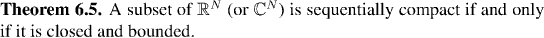Analysis WebNotes
arrow_back arrow_forward

# Class Contents

## Sequentially Compact Sets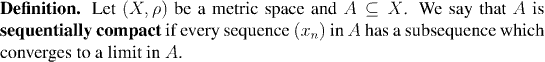So, in other words, what we have seen in Corollary 6.3 is that closed and bounded subsets of the real numbers are sequentially compact. The converse is true, in any metric space: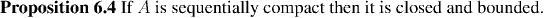In fact, although it is fairly intuitive what is meant, we have not yet given a formal definition of "boundedness" for subsets of a metric space. Because we need it in the present proposition, we now give that definition.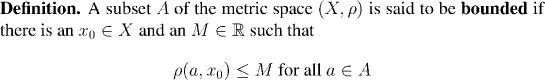Remark:

Although boundedness of a set seems to depend on the choice of x0, it does not. If it is possible to find one x0 and a corresponding M to meet the condition of boundedness, then for every other x in X, we can always find an M which satisfies the conditions.

Thus, Corollary 6.3 and Proposition 6.4 tell us that a set of real numbers is sequentially compact if and only it is closed and bounded. In general, a set can be closed and bounded, but not sequentially compact:

Example: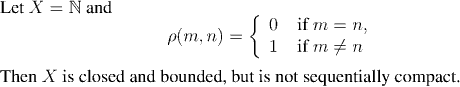However this situation is less typical than one might fear. In most cases, it does turn out that closed and bounded sets are sequentially compact. (You might suspect this, since it took a fairly bizarre example to find a set which is closed and bounded but not sequentially compact!) The following theorem will cover a huge number of situations: